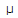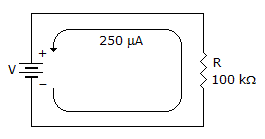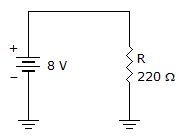# Electronics - Ohm's Law

### Exercise :: Ohm's Law - Filling the Blanks

1.

If the voltage across a resistor ___, the current flowing through it will increase.

 A. increases B. decreases C. stays the same D. Not enough information

Answer: Option A

Explanation:

No answer description available for this question. Let us discuss.

2.

The voltage across a ___ resistor is 12 V and the current through it is 500A.

 A. 600B. 2.4 kC. 6 kD. 24 kAnswer: Option D

Explanation:

No answer description available for this question. Let us discuss.

3.The voltage supplying the current in the given circuit is _____.

 A. 2.5 V B. 25 V C. 250 V D. 2.5 kV

Answer: Option B

Explanation:

V = IR

= 250 x 10^-6 x 100 x 10^3

= 250 x 100 x 10^(-6+3)

= 250 x 100 x 10^-3

= 25 x 1000 x 10^-3

= 25 x 10^3 x 10^-3

= 25 x 10^0

= 25 x 1 [Anything power 0 is 1]

= 25 volts.

4.

The voltage across a 2.2 kresistor is ___ if 3 mA of current is flowing through it.

 A. 6.6 V B. 7.33 V C. 1.4 V D. 0.8 V

Answer: Option A

Explanation:

No answer description available for this question. Let us discuss.

5.The power rating for the resistor in the given circuit should be at least ___.

 A. 1/4 W B. 1/2 W C. 1 W D. 2 W

Answer: Option B

Explanation:

No answer description available for this question. Let us discuss.

#### Current Affairs 2021

Interview Questions and Answers